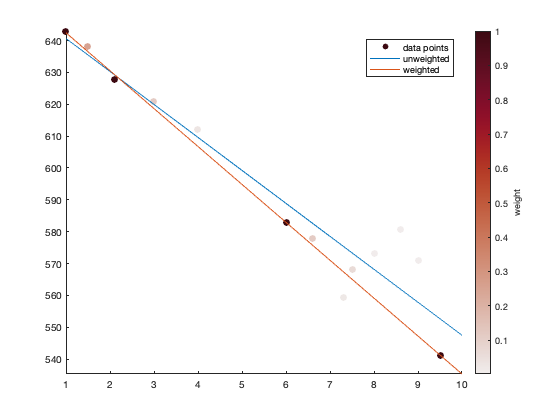# polyfitw documentation

The polyfitw function computes weighted polyfits.

Back to Climate Data Tools Contents. polyfitw computes weighted polyfits.

## Syntax

```p = polyfitw(x,y,n)
p = polyfitw(x,y,n,w)
[p,S,mu] = polyfitw(...)```

## Description

p = polyfitw(x,y,n) calculates the unweighted polynomial fit of x vs y to the nth order, exactly like the standard Matlab polyfit function.

p = polyfitw(x,y,n,w) specifies weights to apply to each y value. For measurements whose formal error estimates are given by err, try using weights w = 1/err.^2.

[p,S,mu] = polyfitw(...) returns the S structure and centering/scaling values mu for use in polyval. Don't forget, if you return more than one output (meaning [p,S,mu] instead of just p), the values in p will be scaled according to the values in mu.

## Example: 1st order:

Here's some scattered data with a known slope of -12, and some prescribed errors associated with each measurement:

```x = [1 1.5 2.1 3 4 6 6.6 7.3 7.5 8 8.6 9 9.5];
err = [1 2 -1 3 6 1 3 -7 4 15 30 25 1];

y = 654 - 12*x + err;

% Weights from errors:
w = 1./err.^2;

figure
scatter(x,y,50,w,'filled')
hold on
axis tight
cb = colorbar;
ylabel(cb,'weight')
cmocean amp
```Here's how to use the standard Matlab function polyfit to find the unweighted slope of the line

```p = polyfit(x,y,1)
```
```p =
-10.35        650.99
```

That tells us the unweighted slope is -10.35, although we imposed a slope of -12. The difference is due to the error in the measurements. Notably, you can use the CDT trend function to get the same answer:

```trend(y,x)
```
```ans =
-10.35
```

The mismatch between the -10.35 slope value and the imposed -12 slope is due to measurement error. Fortunately, we know how much weight to give to each measurement, and we can weight accordingly with polyfitw:

```pw = polyfitw(x,y,1,w)
```
```pw =
-11.90        654.34
```

Now -11.9 is not exactly the -12 slope we imposed, but this is much closer than the estimate from the unweighted trend. Here's the difference:

```xi = 1:10;

hold on
plot(xi,polyval(p,xi))
plot(xi,polyval(pw,xi))

legend('data points','unweighted','weighted')
```## Author Info

This function is part of the Climate Data Toolbox for Matlab. The function and supporting documentation were written by Chad A. Greene of NASA Jet Propulsion Laboratory.